Categories: Quantum information.

Repetition code

A repetition code is a simple approach to error correction: to protect a bit $$x$$, make two copies:

\begin{aligned} 0 \to 000 \qquad \quad 1 \to 111 \end{aligned}

If a single-bit error occurs, e.g. $$000 \to 100$$, a majority vote resets the minority bit. Clearly, this does not protect against multi-bit errors, but that is usually not necessary.

In quantum computing, where error correction is much more important, repetition codes can also be used, albeit with some complications, as discussed below.

Bit flip code

Suppose that we want to detect errors in the following arbitrary qubit state $$\ket{\psi}$$:

\begin{aligned} \ket{\psi} = \alpha \ket{0} + \beta \ket{1} \end{aligned}

For now, let us limit ourselves to detecting bit flips, where $$\alpha$$ and $$\beta$$ get switched:

\begin{aligned} \alpha \ket{0} + \beta \ket{1} \quad \to \boxed{\mathrm{Error}} \to \quad \beta \ket{0} \!+\! \alpha \ket{1} \end{aligned}

One way to defend against this is the quantum version of a classical repetition code:

\begin{aligned} \ket{\psi} \quad \to \boxed{\mathrm{Encoder}} \to \quad \ket*{\overline{\psi}} = \alpha \ket{000} + \beta \ket{111} \end{aligned}

In other words, a logical $$\ket{0}$$ (written $$\ket*{\overline{0}}$$) is represented by 3 physical qubits, and vice versa:

\begin{aligned} \boxed{ \ket{0} \to \ket*{\overline{0}} = \ket{000} \qquad \quad \ket{1} \to \ket*{\overline{1}} = \ket{111} } \end{aligned}

Such a transformation is easy to achieve with the following sequence of quantum gates: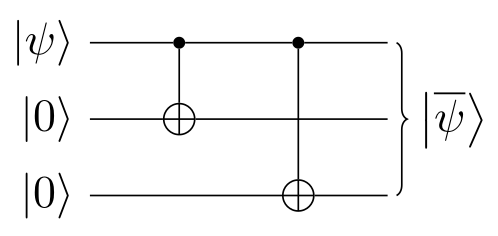So, a little while after encoding the state $$\ket{\psi}$$ like that, a bit flip occurs on the 2nd qubit:

\begin{aligned} \ket*{\overline{\psi}} \quad \to \boxed{\mathrm{Error}} \to \quad \alpha \ket{010} + \beta \ket{101} \end{aligned}

But now there is a problem: how do we detect this error? We could measure the state, but that would make it collapse, which is probably not what we want.

The trick is to use operators called stabilizers, in this case for example $$ZZI = Z_1 \otimes Z_2 \otimes I_3$$, where $$I$$ is identity and $$Z$$ is the Pauli-$$Z$$ gate. The 3-qubit basis states are its eigenvectors:

\begin{alignedat}{2} ZZI \ket{000} &= + \ket{000} \qquad ZZI \ket{001} &&= + \ket{001} \\ ZZI \ket{010} &= - \ket{010} \qquad ZZI \ket{011} &&= - \ket{011} \\ ZZI \ket{100} &= - \ket{100} \qquad ZZI \ket{101} &&= - \ket{101} \\ ZZI \ket{110} &= + \ket{110} \qquad ZZI \ket{111} &&= + \ket{111} \end{alignedat}

We could measure $$ZZI$$ for $$\ket*{\overline{\psi}}$$, and if the eigenvalue is $$-1$$, we know that a bit flip has occurred, whereas if the eigenvalue is $$+1$$, there is maybe no error ($$\ket{001}$$ and $$\ket{110}$$ are false negatives).

These false negatives are fixed by including another stabilizer $$IZZ$$, with these eigenvectors:

\begin{alignedat}{2} IZZ \ket{000} &= + \ket{000} \qquad IZZ \ket{001} &&= - \ket{001} \\ IZZ \ket{010} &= - \ket{010} \qquad IZZ \ket{011} &&= + \ket{011} \\ IZZ \ket{100} &= + \ket{100} \qquad IZZ \ket{101} &&= - \ket{101} \\ IZZ \ket{110} &= - \ket{110} \qquad IZZ \ket{111} &&= + \ket{111} \end{alignedat}

In which case $$\ket{100}$$ and $$\ket{011}$$ are false negatives. In other words, $$IZZ$$ cannot detect if the 1st qubit was flipped, while $$ZZI$$ cannot protect the 3rd qubit. But by using both, we know exactly which qubit was flipped thanks to the eigenvalues:

Error $$ZZI$$ $$IZZ$$
$$I$$ $$+1$$ $$+1$$
$$X_1$$ $$-1$$ $$+1$$
$$X_2$$ $$-1$$ $$-1$$
$$X_1$$ $$+1$$ $$-1$$

Where e.g. $$X_3$$ denotes that the 3rd qubit was flipped. The measurement outcomes on the last three rows are called error syndromes, and are obtained by a syndrome measurement.

Fortunately, we can measure $$ZZI$$ and $$IZZ$$ without affecting $$\ket*{\overline{\psi}}$$ itself, by applying $$\mathrm{CNOT}$$s to some ancillary qubits and then measuring those: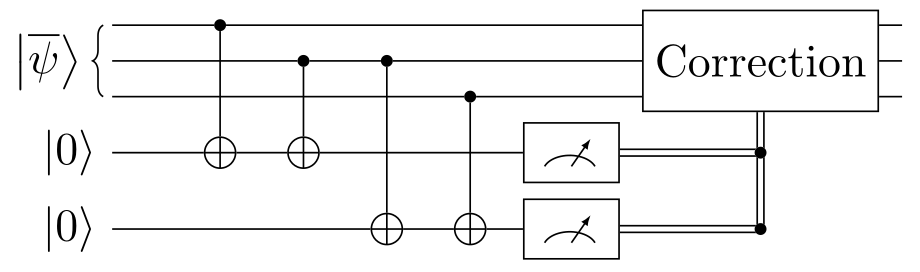The two measurements, respectively representing $$ZZI$$ and $$IZZ$$, yield $$\ket{1}$$ if a bit flip definitely occurred, and $$\ket{0}$$ otherwise. There is no entanglement, so the input is untouched.

Phase flip code

The above system protects us against all single-qubit bit flips. Unfortunately, that is not enough: qubits can also experience a phase flip:

\begin{aligned} \alpha \ket{0} + \beta \ket{1} \quad \to \boxed{\mathrm{Error}} \to \quad \alpha \ket{0} - \beta \ket{1} \end{aligned}

How to detect that? If we want to protect against phase flips instead of bit flips, we can simply do the same as before, but along the $$X$$-axis intead of the $$Z$$-axis:

\begin{aligned} \boxed{ \ket{0} \to \ket*{\overline{0}} = \ket{+\!+\!+} \qquad \quad \ket{1} \to \ket*{\overline{1}} = \ket{-\!-\!-} } \end{aligned}

Such that an arbitrary state $$\ket{\psi}$$ is encoded as follows, by the circuit shown below:

\begin{aligned} \ket{\psi} \quad \to \boxed{\mathrm{Encoder}} \to \quad \ket*{\overline{\psi}} = \alpha \ket{+\!+\!+} + \beta \ket{-\!-\!-} \end{aligned}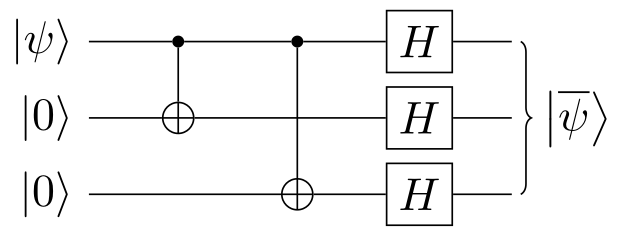A phase flip along the $$Z$$-axis corresponds to a bit flip along the $$X$$-axis $$\ket{+} \to \ket{-}$$. In this case, the stabilizers are $$XXI$$ and $$IXX$$, and the error detection circuit is as follows: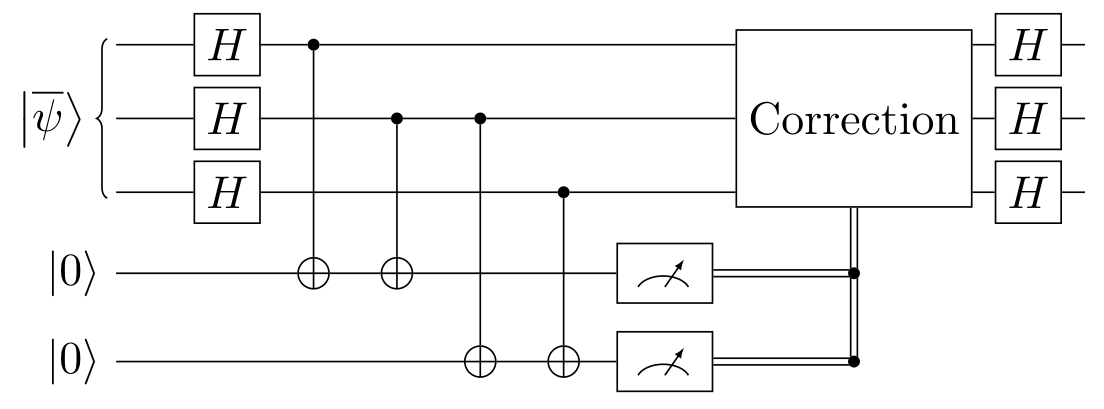This system protects us against all single-qubit phase flips, but not against bit flips.

Shor code

What kind of repetition code would we need if we want to detect both bit flips and phase flips? The most straightforward option is the Shor code. Starting from a phase flip encoding:

\begin{aligned} \ket{0} \to \ket*{\overline{0}} &= \ket{+\!+\!+} = \bigg( \frac{\ket{0} + \ket{1}}{\sqrt{2}} \bigg)^{\otimes 3} \\ \ket{1} \to \ket*{\overline{1}} &= \ket{-\!-\!-} = \bigg( \frac{\ket{0} - \ket{1}}{\sqrt{2}} \bigg)^{\otimes 3} \end{aligned}

We add protection against bit flips by using a repetition code for each physical qubit:

\begin{aligned} \boxed{ \ket*{\overline{0}} = \bigg( \frac{\ket{000} + \ket{111}}{\sqrt{2}} \bigg)^{\otimes 3} \qquad \quad \ket*{\overline{1}} = \bigg( \frac{\ket{000} - \ket{111}}{\sqrt{2}} \bigg)^{\otimes 3} } \end{aligned}

This encoding is achieved by the following quantum circuit, which simply consists of the phase flip encoder, followed by 3 copies of the bit flip encoder: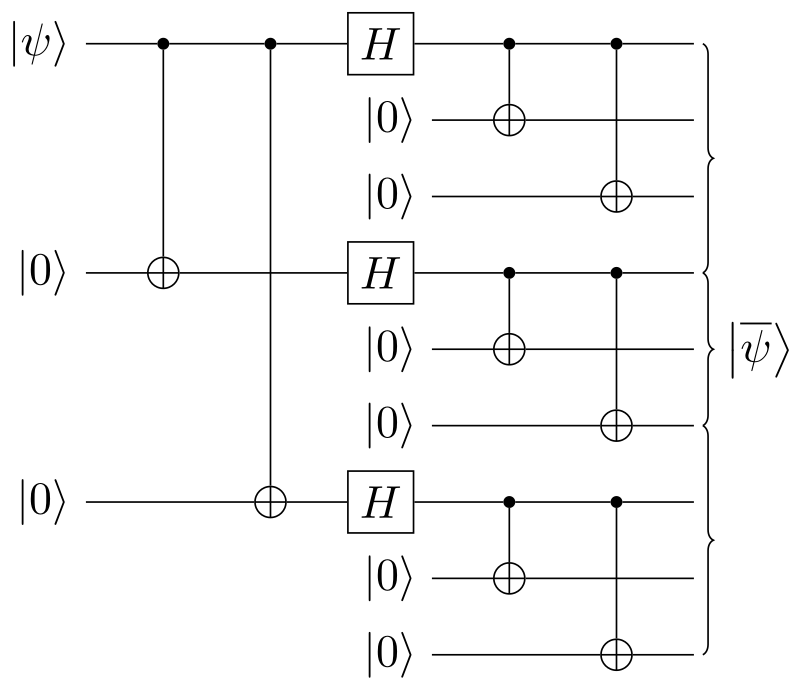We thus use 9 physical qubits to store 1 logical qubit. Fortunately, more efficient schemes exist.

The bit flip stabilizers $$ZZI$$ and $$IZZ$$ are applied on a per-block basis, like so:

\begin{aligned} ZZI \: III \: III \qquad\quad III \: ZZI \: III \qquad\quad III \: III \: ZZI \\ IZZ \: III \: III \qquad\quad III \: IZZ \: III \qquad\quad III \: III \: IZZ \end{aligned}

Whereas the phase flip stabilizers $$XXI$$ and $$IXX$$ are applied to entire blocks at once:

\begin{aligned} XXX \: XXX \: III \qquad \quad III \: XXX \: XXX \end{aligned}

1. J.S. Neergaard-Nielsen, Quantum information: lectures notes, 2021, unpublished.
2. S. Aaronson, Introduction to quantum information science: lecture notes, 2018, unpublished.

© Marcus R.A. Newman, a.k.a. "Prefetch". Available under CC BY-SA 4.0.